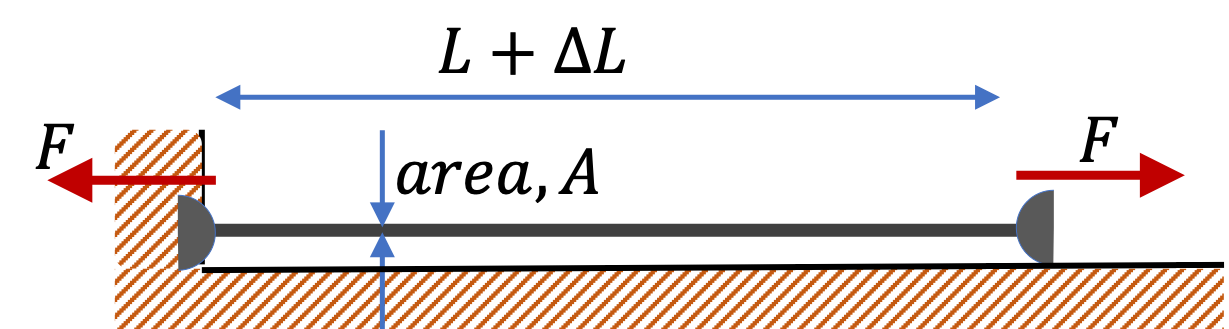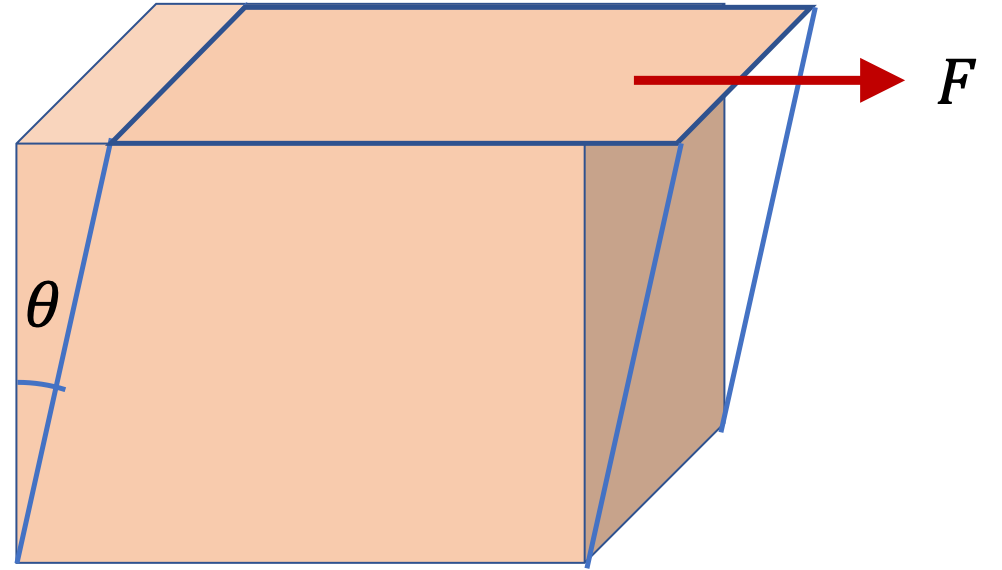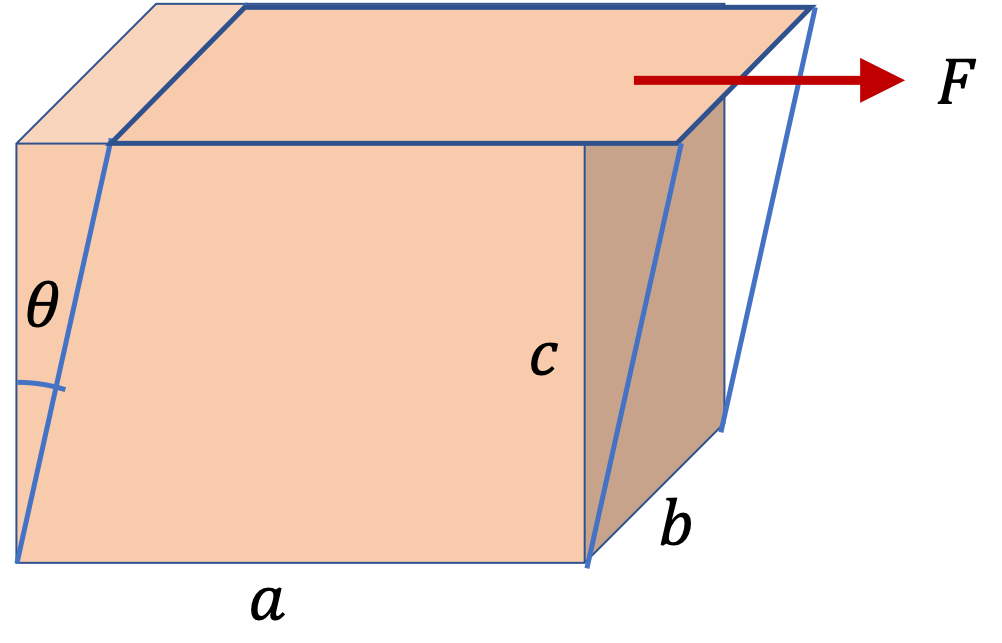## Section16.4Energy in Strained Material

If strain in a matrial is not too great, the material will go back to the original shape when stress-causing force is removed. The energy stored in the strained material in the elastic region is just like the energy of a spring and is called elastic energy.

When you strecth a wire by pulling on it by some force at the ends as in Figure 16.4.1, the quantity $Y A/L$ acts similar to spring constant of a spring.

\begin{equation*} k \equiv Y\dfrac{A}{L}, \end{equation*}

with the stretching $\Delta L$ to be the $x$ of spring.

\begin{equation*} x \equiv \Delta L, \end{equation*}

and strain will be

\begin{equation*} \epsilon = \dfrac{\Delta L}{L} = \dfrac{x}{L}. \end{equation*}

Therefore, potential energy stored in a strained material, i.e., the elastic energy, will be

\begin{equation*} U = \dfrac{1}{2} k x^2 = \dfrac{1}{2} \dfrac{YA}{L} \left( \Delta L \right)^2 = \dfrac{1}{2}YV\epsioon^2, \end{equation*}

where $V = AL\text{,}$ the volume of the material. This elastic energy is due to the stretching of the wire, therefore, it is also called tensile energy. A similar formula can be obtained for compressive elastic energy. Dividing by the volume of the wire, we see that elastic energy density, i.e., elastic energy per unit volume depends only on the elastic modulus and strain.

\begin{equation*} u = \dfrac{U}{V} = \dfrac{1}{2} Y \, \epsilon^2. \end{equation*}Figure 16.4.1. The elastic energy stored in the stretched wire is $\dfrac{1}{2} YV \epsilon^2\text{,}$ with $\epsilon = \Delta L/L\text{.}$

When you look at a volume deformation, the strain is

\begin{equation*} \epsilon = \dfrac{\Delta V}{V}, \end{equation*}

and the modulus is the bulk modulus, $B\text{.}$ The elastic energy stored in volume strain is

\begin{equation} U = \frac{1}{2}B V \epsilon^2.\tag{16.4.1} \end{equation}

Similarly, the energy stored in shear strain $\theta$ is

\begin{equation} U = \frac{1}{2}V G \theta^2,\tag{16.4.2} \end{equation}

where $G$ is the shear modulus.From different formulas for different types of deformation, you can guess a common formula that you can apply to any type.

\begin{equation} U = \dfrac{1}{2} V \times \text{Modulus} \times \text{Strain}^2.\tag{16.4.3} \end{equation}

A $100\text{-kg}$ steel ball is hung from a high ceiling using a $8\text{-m}$ long steel cable of diameter $4.0\text{mm}\text{.}$ How much elastic energy is stored in the cable? Data: Young's modulus of steel = $220\text{ GPa}\text{.}$

Hint

Use formula after finding the strain.

$1.39\text{ J} \text{.}$

Solution

First we find the strain $\Delta L/L$ by Hooke's law. For that we need to work out stress, for which we need the area of cross-section.

\begin{gather*} A = \pi R^2 = \pi \times \left( 2\times 10^{-3} \right)^2 = 1.25\times 10^{-5}\text{ m}^2 \\ F = mg = 100\times 9.81 = 981\text{ N} \end{gather*}

Therefore, stress in the cable is

\begin{equation*} \text{Stress } = \dfrac{F}{A} = 7.8\times 10^7\text{ N/m}^2. \end{equation*}

Using $Y = 220\text{ GPa} = 220\times 10^9\text{ Pa}\text{,}$ we can get the strain caused in the cable.

\begin{equation*} \dfrac{\Delta L}{L} = \dfrac{1}{Y} \dfrac{F}{A} = 3.55\times 10^{-4}. \end{equation*}

Therefore, the tensile energy stored in the cable is

\begin{align*} U \amp = \dfrac{1}{2} YV\left( \dfrac{\Delta L}{L} \right)^2,\\ \amp = \dfrac{1}{2} 220\times 10^9 \times 1.25\times 10^{-5} \times 8\times \left( 3.55\times 10^{-4} \right)^2 = 1.39\text{ J}. \end{align*}

The Achiles tendon contracts and stretches as you run. Suppose a $80\text{-kg}$ man has $10\text{-cm}$ long tendon with area of cross-section $0.75\text{ cm}^2\text{.}$ Data: Young's modulus of tendon = $1.5\text{ GPa}\text{.}$

(a) When you run you tend to strike the ground with considerable more force than just your weight. Suppose at some instant, the force on the tendon of the runner is 5 times his weight and the tendon is compressed by this force. (i) What is the stress in the Achiles tendon at this instant? (ii) What is the strain of the Achiles tendon at this instant?

(b) How much strain energy is stored at the instant in (a)?

Hint

(a) Pay attention to units and use definitions. (b) Assume linear regime.

(a)(i) $5.23\times 10^7\text{ N/m}^2 \text{,}$ (ii) $0.035$ , (b) $6.89\text{ J}\text{.}$

Solution 1 (a)

(a) (i) From the statement we note that a force of 5 times the weight acts on an area $0.75\text{ cm}^2\text{,}$ which will give stress

\begin{equation*} \text{Stress } = \dfrac{5\times 80\times 9.81}{0.75\times 10^{-4}} = 5.23\times 10^7\text{ N/m}^2. \end{equation*}

(ii) With this stress, the strain will be

\begin{equation*} \dfrac{\Delta L}{L} = \dfrac{\text{ Stress}}{Y} = \dfrac{5.23\times 10^7}{1.5\times 10^9} = 0.035. \end{equation*}
Solution 2 (b)

(b) Assuming linear regime, we would have the following amount of energy stored.

\begin{align*} U \amp = \dfrac{1}{2} YV\left( \dfrac{\Delta L}{L} \right)^2\\ \amp = \dfrac{1}{2} 1.5\times 10^9 \times (0.75 \times 10^{-4} \times 0.10) \times 0.035^2 = 6.89\text{ J}. \end{align*}

A rectangular parallelepiped-shaped jello with dimensions $(a\times b \times c) = 5\text{ cm} \times 4\text{ cm} \times 3\text{ cm}$ is resting on a plate. A glass slide is glued to the top surface. A force of $F = 30\text{ mN}$ is applied to the glass plate deforming the jello sideways.

What is the amount of elastic energy stored in the jello?

Data: shear modulus of $G=1000\text{ Pa}\text{.}$Hint

Find strain and then use formula for energy.

$4.5\times 10^{-4} \text{ J}\text{.}$

Solution

From Checkpoint 16.3.7 we have the shear strain to be

\begin{equation*} \theta = 1.5\times 10^{-2}\text{ rad}. \end{equation*}

The volume of undeformed jello is

\begin{equation*} V = 5\text{ cm} \times 4\text{ cm} \times 3\text{ cm} = 60\times 10^{-6}\text{ m}^3. \end{equation*}

Therefore, the stored energy is

\begin{align*} U \amp = \dfrac{1}{2}\times 1000\text{ Pa} \times 60\times 10^{-6} \text{ m}^3 \times 1.5\times 10^{-2} \text{ rad},\\ \amp = 4.5\times 10^{-4} \text{ J}. \end{align*}